# Problem # 1 Determine ly and ly for the section given below 120 mm 400 mmm...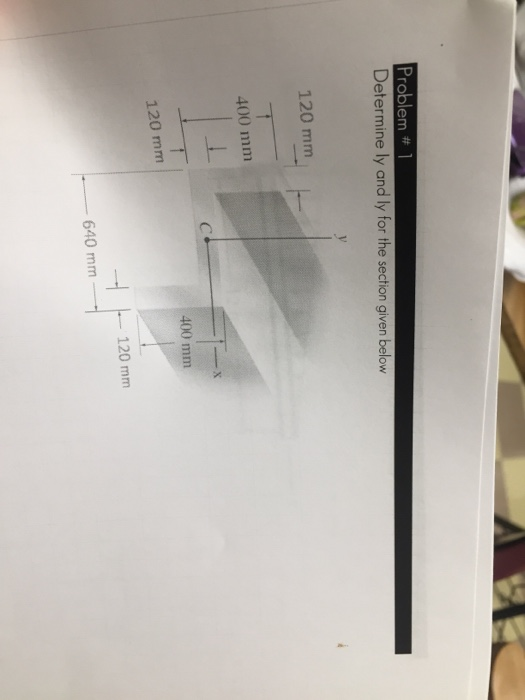Problem # 1 Determine ly and ly for the section given below 120 mm 400 mmm 400 mm 120 mm -120 mm 640 mm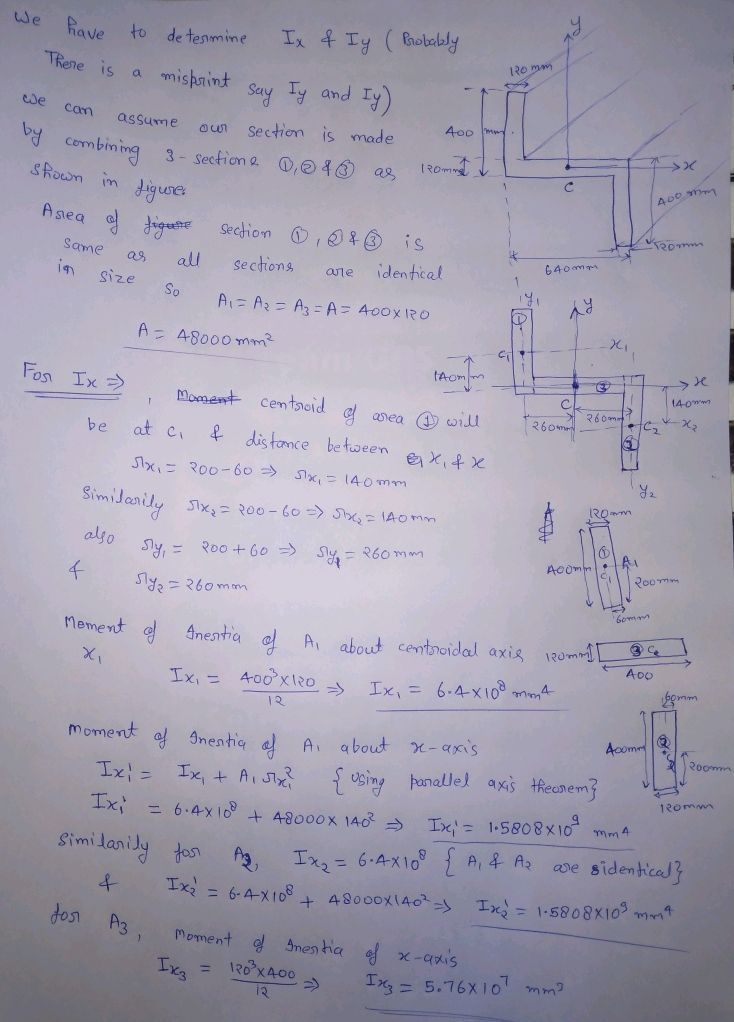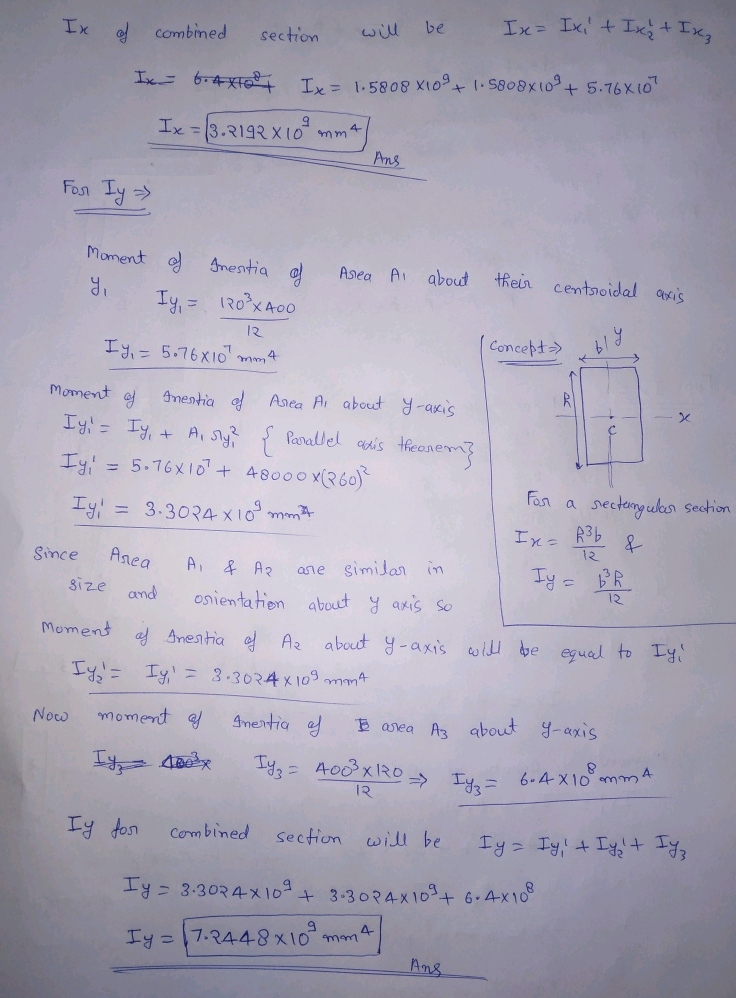##### Add Answer to: Problem # 1 Determine ly and ly for the section given below 120 mm 400 mmm...
Similar Homework Help Questions
• ### 2. Determine ly and ky. Hint: Section 1 is the left triangle. Section 2 is the...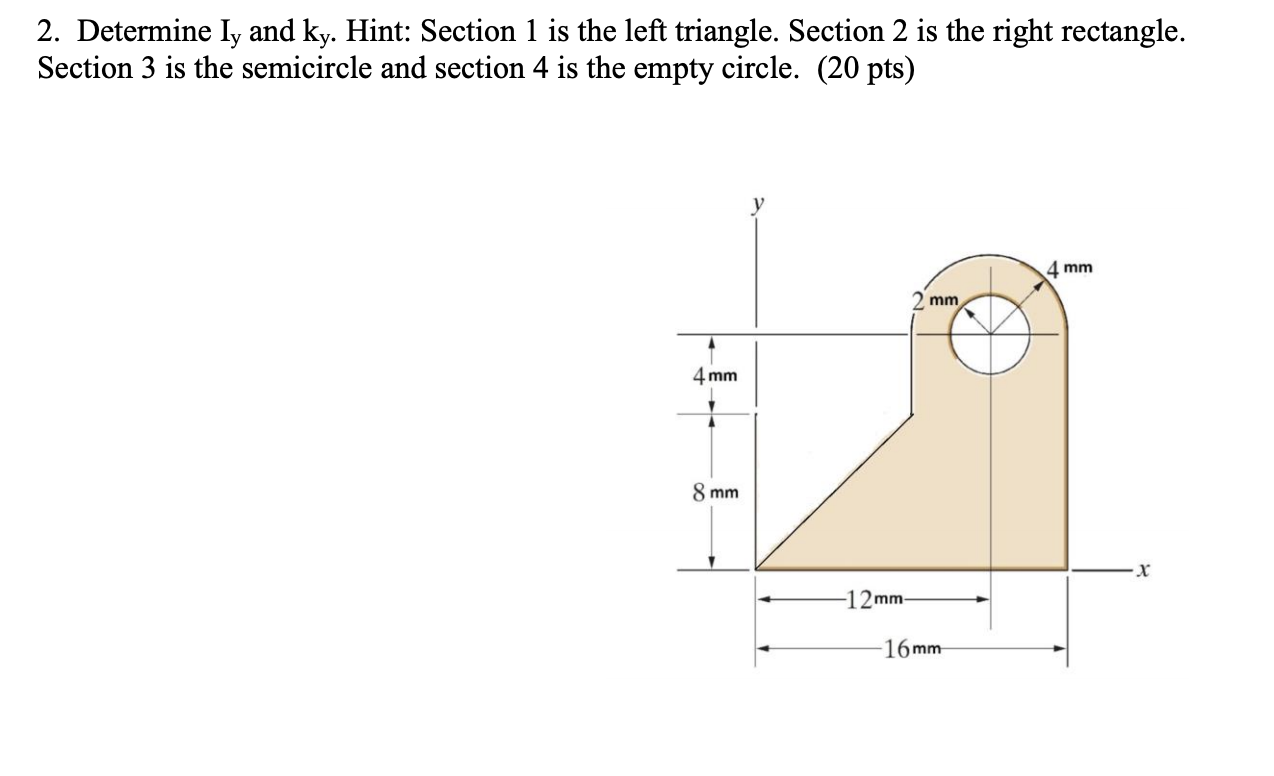2. Determine ly and ky. Hint: Section 1 is the left triangle. Section 2 is the right rectangle. Section 3 is the semicircle and section 4 is the empty circle. (20 pts) у 4 mm mm 4 mm 1 8 mm X -12mm- 16 mm

• ### 2. Determine ly and ky. Hint: Section 1 is the left triangle. Section 2 is the...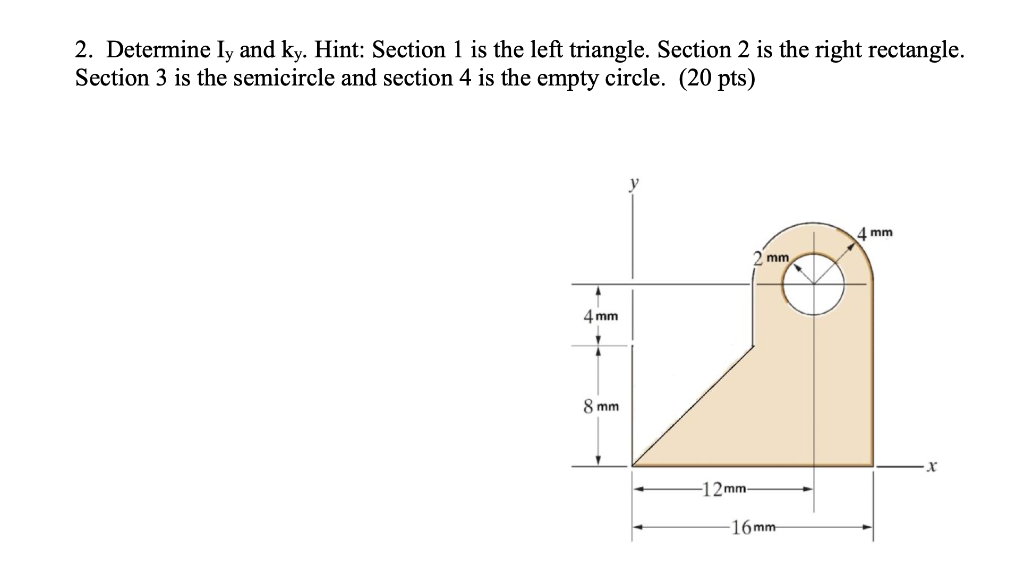2. Determine ly and ky. Hint: Section 1 is the left triangle. Section 2 is the right rectangle. Section 3 is the semicircle and section 4 is the empty circle. (20 pts) 4 mm mm 4mm 8 mm -12mm 16 mm

• ### 2. Determine ly and ky. Hint: Section 1 is the left triangle. Section 2 is the...2. Determine ly and ky. Hint: Section 1 is the left triangle. Section 2 is the right rectangle. Section 3 is the semicircle and section 4 is the empty circle. (20 pts) 4 mm mm 4mm 8 mm -12mm 16 mm

• ### PROBLEM 2: 40% A 6 kN force is exerted on the frame which has the T cross sectio analyze the states of stress at a section taken at 800 mm from the point of n shown below. It is required to 1. Fo...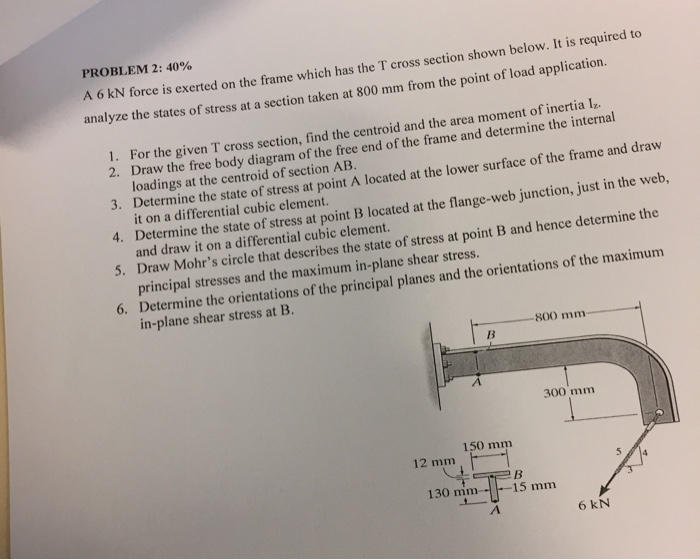PROBLEM 2: 40% A 6 kN force is exerted on the frame which has the T cross sectio analyze the states of stress at a section taken at 800 mm from the point of n shown below. It is required to 1. For the given T cross section, find the centroid and the area moment of inertia I,. 2. Draw the free body diagram of the free end of the frame and determine the interna loadings at the centroid of...

• ### QUESTION 1 Given the dimension a = 176 mm and the applied load is 400 N,...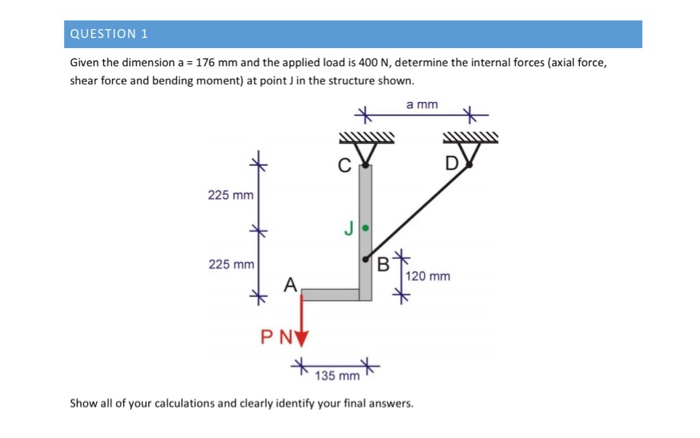QUESTION 1 Given the dimension a = 176 mm and the applied load is 400 N, determine the internal forces (axial force, shear force and bending moment) at point in the structure shown. a mm WW 225 mm * 225 mm R * 120 mm A * PNV * 135 mm* Show all of your calculations and clearly identify your final answers.

• ### Determine the beam's moment of inertia ly about the centroidal y axis. 15 mm 15 mm...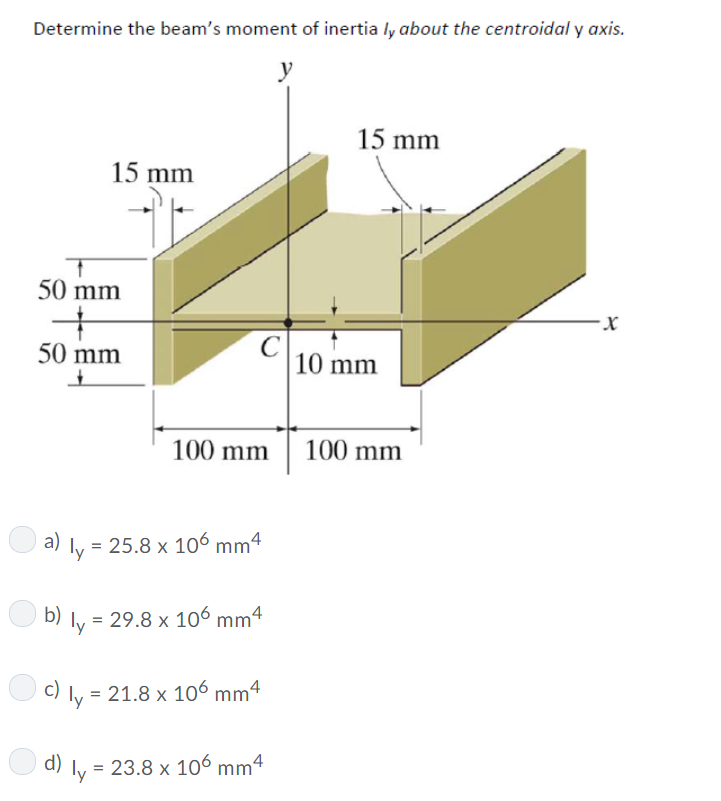Determine the beam's moment of inertia ly about the centroidal y axis. 15 mm 15 mm 50 mm X C 10 mm 50 mm 100 mm 100 mm a) ly 25.8 x 106 mm4 = b) ly 29.8 x 106 mm4 c) Iv = 21.8 x 100 mm4 d) 23.8 x 106 mm4 Determine the beam's moment of inertia ly about the centroidal y axis. 15 mm 15 mm 50 mm X C 10 mm 50 mm 100 mm 100...

• ### Problem 2 Determine the centroid of the shaded area. 40 mm 20 mm 80 mm 120...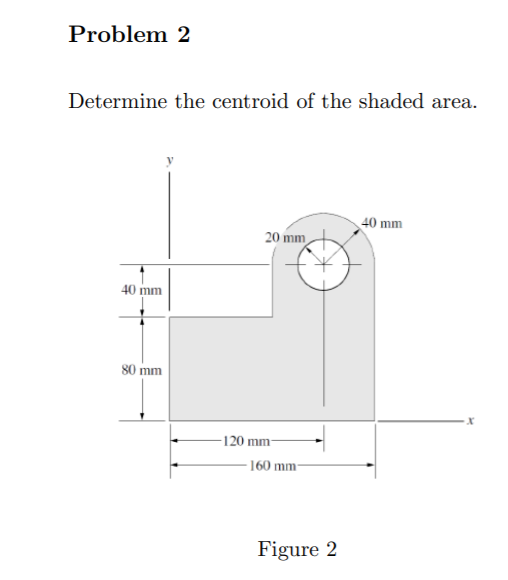Problem 2 Determine the centroid of the shaded area. 40 mm 20 mm 80 mm 120 mm 160 mm Figure 2

• ### 冘. 100 mm 400 mm 57.1M 20 kN-m 100 mm 300 mm Figure 2. Determine the bending stress at Points A ...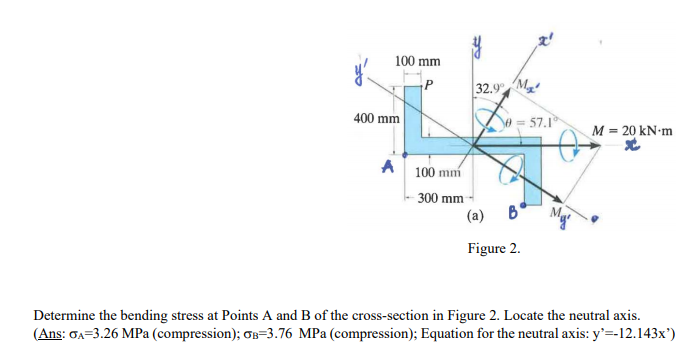冘. 100 mm 400 mm 57.1M 20 kN-m 100 mm 300 mm Figure 2. Determine the bending stress at Points A and B of the cross-section in Figure 2. Locate the neutral axis. ( Ans: ơ,-326 MPa (compression); ơB-376 MPa (compression): Equation for the neutral axis: y'--12.143 x') 冘. 100 mm 400 mm 57.1M 20 kN-m 100 mm 300 mm Figure 2. Determine the bending stress at Points A and B of the cross-section in Figure 2. Locate the neutral...

• ### Question 1 (4 marks): Determine the nominal member capacity (Ne) of a steel column with the following heavily welded cross-section. The column has an effective length of 4.7 m and a yield stress of 3...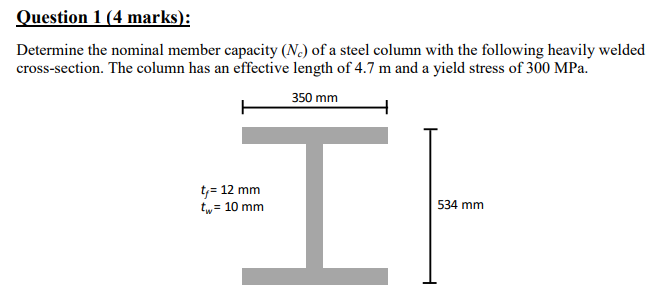Question 1 (4 marks): Determine the nominal member capacity (Ne) of a steel column with the following heavily welded cross-section. The column has an effective length of 4.7 m and a yield stress of 300 MPa. 350 mmm t 12 mm tw 10 mm 534 mm Question 1 (4 marks): Determine the nominal member capacity (Ne) of a steel column with the following heavily welded cross-section. The column has an effective length of 4.7 m and a yield stress of...

• ### Question 4: For the Figure below determine the location of the centroid (x,y). 150 mm 150...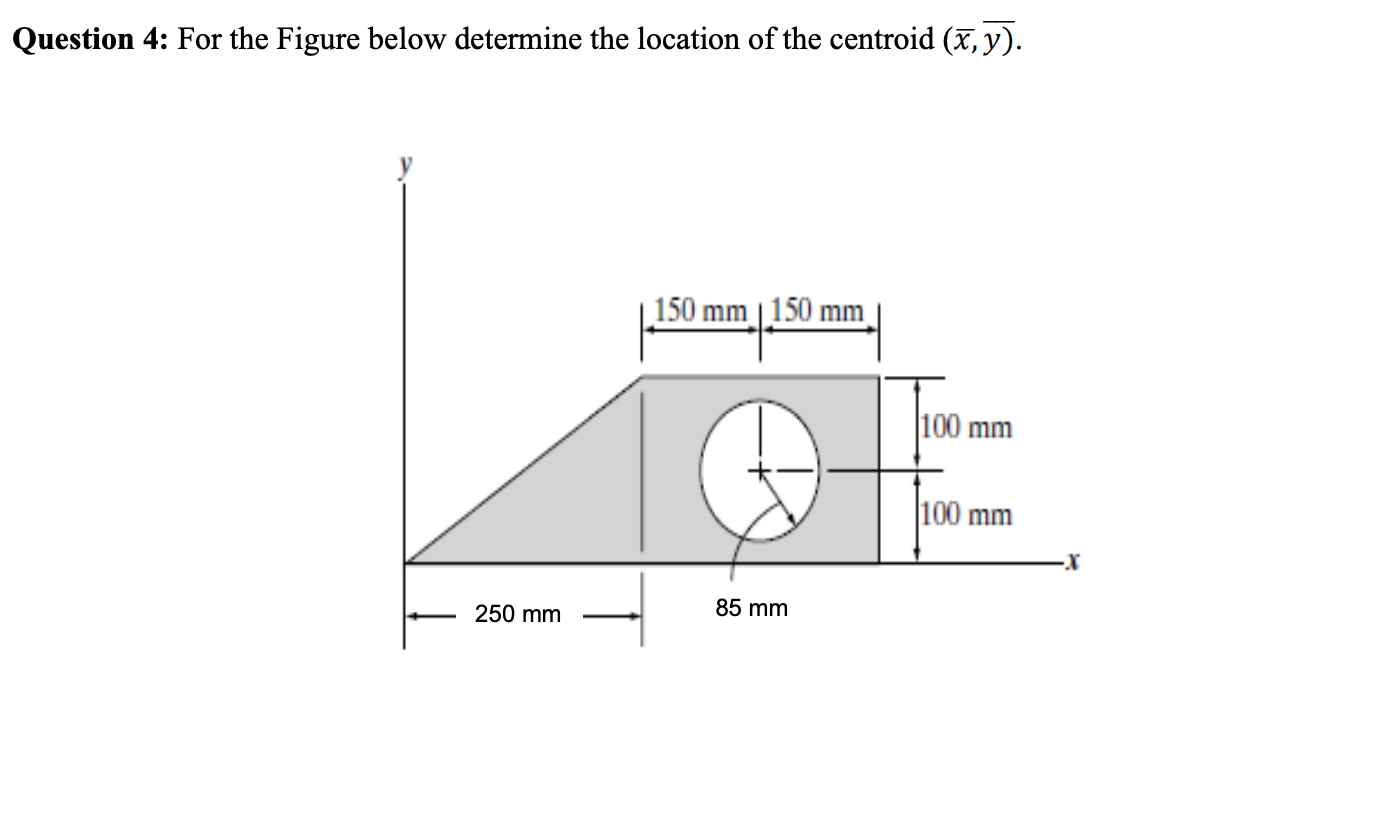Question 4: For the Figure below determine the location of the centroid (x,y). 150 mm 150 mm 100 mm 100 mm 250 mm 85 mm Question 3: For the Figure below determine the location of the centroid (,y). 108 mm 36 mm| 24 mm 400 mm X 48 mm 150 mm Question 2: Figure below is symmetrical about the vertical Y axis. Calculate the distance of the centre of area (centroid) of cross section from the base. 0.5 in. 0.5...

Free Homework App